Triangles in Squares

The following pictures show n equilateral triangles with side 1 packed inside the smallest known square (of side length s).

 1.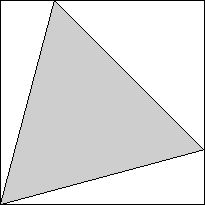2.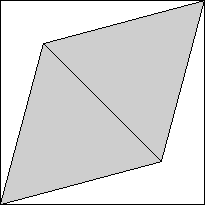3.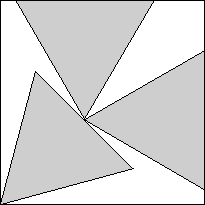s = (√2 + √6) / 4 = .965+Trivial. s = √6/2 = 1.224+Trivial. s = √3/2 + √6/4 = 1.478+Found by Erich Friedmanin 1996.

 4.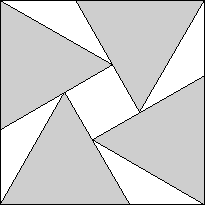5.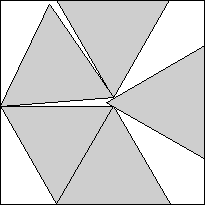6.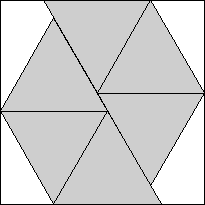s = 1 + 1 / √3 = 1.577+Found by Erich Friedmanin 1996. s = 1.803+Found by Erich Friedmanin 1996. s = 9 / 2 - 3 √3 / 2 = 1.901+Found by Erich Friedmanin 1996.

 7.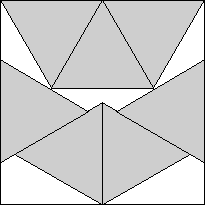8.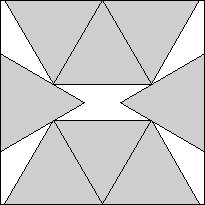9.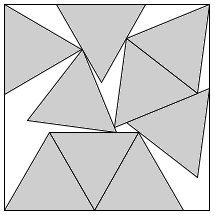s = 2Found by Erich Friedmanin 1996. s = 3 √3 / 2 - 1 / 2 = 2.098+Found by Erich Friedmanin 1996. s = 2.287+Found by Maurizio Morandiin July 2008.

 10.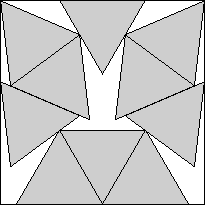11.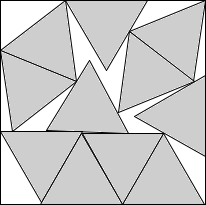12.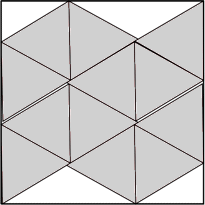s = 2.377+Found by David W. Cantrellin July 2002. s = 2.490+Found by Maurizio Morandiin June 2008. s = 7√3/2 - 7/2 = 2.562+Found by David W. Cantrellin July 2002.

 13.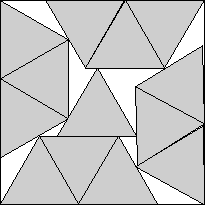14.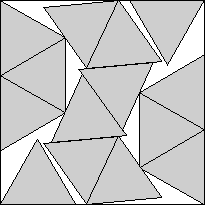15.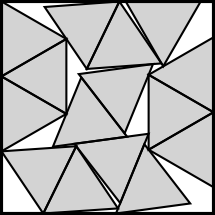s = 2.595+Found by David W. Cantrellin July 2002. s = 2.726+Found by David W. Cantrellin July 2002. s = 2.830+Found by Maurizio Morandiin July 2008.

 16.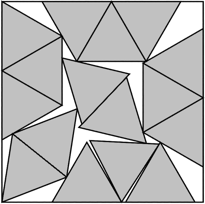17.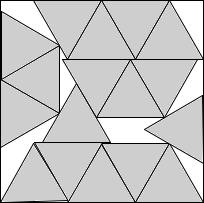18.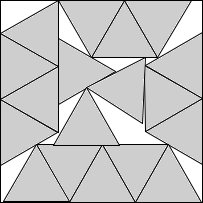s = 2.900+Found by Maurizio Morandiin July 2008. s = 2.982+Found by Maurizio Morandiin July 2008. s = 3.051+Found by Maurizio Morandiin July 2008.

 19.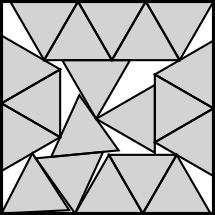20.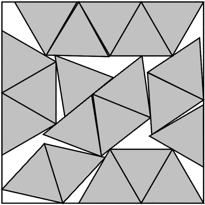21.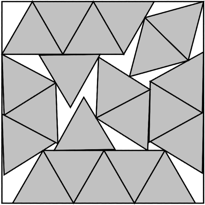s = 4 - √3 / 2 = 3.133+Found by Maurizio Morandiin July 2008. s = 3.233+Found by Károly Hajbain August 2008. s = 10√3 - 14 = 3.320+Found by Maurizio Morandiin July 2008.

 22.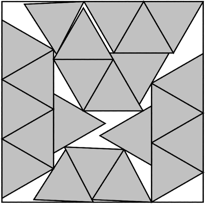s = 3.378+Found by David W. Cantrellin August 2012. 23.s = 3.436+Found by Maurizio Morandiin August 2008. 24.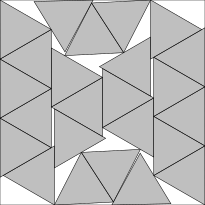s = 3.468+Found by Maurizio Morandiin August 2008.

 25.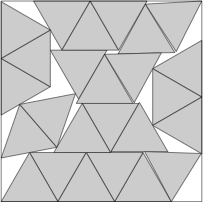s = 3.537+Found by David W. Cantrellin August 2012. 26.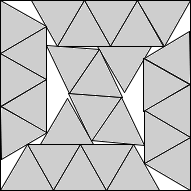s = 3.575+Found by Maurizio Morandiin September 2008. 27.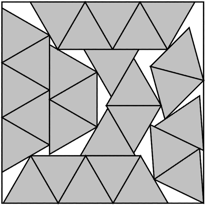s = 3.675+Found by Maurizio Morandiin September 2008.